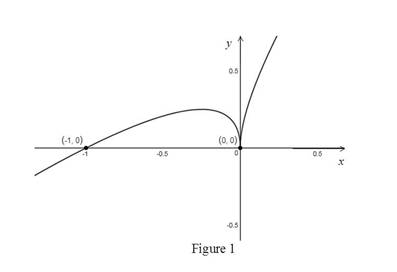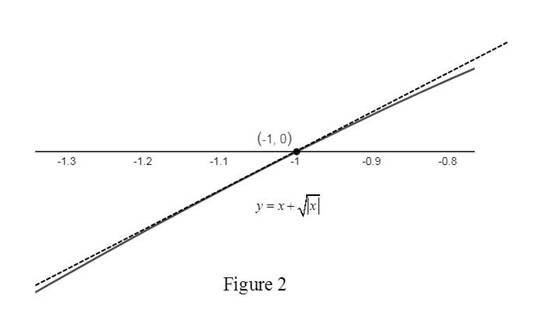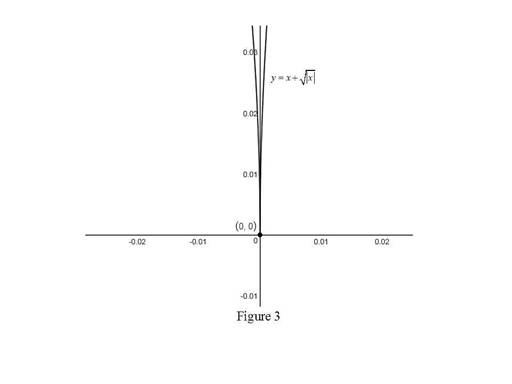# To graph: The function f ( x ) = x + | x | , and conclude the differentiability of the points ( − 1 , 0 ) and ( 0 , 0 ) .### Single Variable Calculus: Concepts...

4th Edition
James Stewart
Publisher: Cengage Learning
ISBN: 9781337687805### Single Variable Calculus: Concepts...

4th Edition
James Stewart
Publisher: Cengage Learning
ISBN: 9781337687805

#### Solutions

Chapter 2.7, Problem 39E
To determine

## To graph: The function f(x)=x+|x|, and conclude the differentiability of the points (−1,0) and (0,0).

Expert Solution

The function f(x) is differentiable at (1,0) but not differentiable at (0,0).

### Explanation of Solution

Given:

The given function is f(x)=x+|x|.

Graph:

Use the online graphing calculator to draw the graph of the function f(x)=x+|x| as shown below in Figure 1.Check the behavior zoom in the graph toward the point (1,0).

Graph:

Use the online graphing calculator to zoom toward the point (1,0) as shown below in Figure 2.Observation:

From Figure 2, it is observed that when zoomed in the graph toward the point (1,0), the graph of the tangent line and the curve looks likes almost identical.

Hence, the given function f(x)=x+|x| is differentiable at (1,0).

Check the behavior zoom in the graph toward the point (0,0).

Graph:

Use the online graphing calculator to zoom toward the point (0,0) as shown below in Figure 3.Observation:

From Figure 3, it is observed that when zoomed in the graph toward the point (0,0), the curve has high sharpness at origin. So, there is no unique tangent line at origin.

Thus, origin is a corner point.

Hence, the given function f(x)=x+|x| is not differentiable at (0,0).

Conclusion:

By zooming the graph toward the point (1,0), the graph of the tangent line and the curve looks likes almost identical while zooming the graph toward the point (0,0),the graph has no unique tangent line.

Thus, f(x)=x+|x| is differentiable at (1,0) but not differentiable at (0,0).

### Have a homework question?

Subscribe to bartleby learn! Ask subject matter experts 30 homework questions each month. Plus, you’ll have access to millions of step-by-step textbook answers!